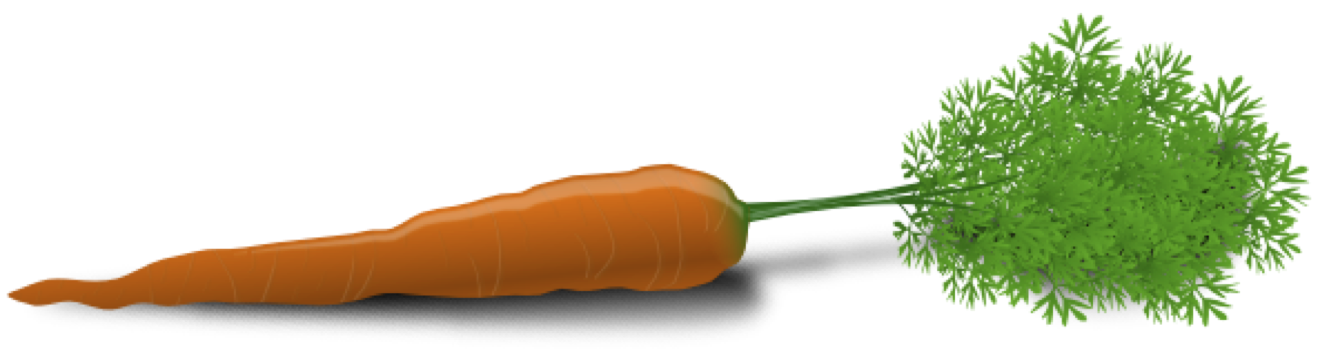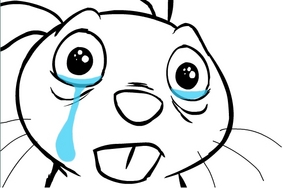# Data Modeling with caret¶

## Classification And REgression Training¶# Part 1: Machine Learning in R Without caret¶## A simple machine learning example that does not take advantage of caret¶

First, we load the required libraries and read our data into data frames. The following data (and most of the code) come from kaggle's introductory "Digit Recognizer" optical character recognition problem.

In :
library(randomForest)
trainData <- read.csv("data/digits/train.csv")
trainData$label <- as.factor(trainData$label)
testData <- read.csv("data/digits/test.csv")


Let's verify that the data look properly loaded, and inspect the format.

In :
dim(trainData); dim(testData); str(trainData[, 1:6])

Out:
1. 42000
2. 785
Out:
1. 28000
2. 784
'data.frame':	42000 obs. of  6 variables:
$label : Factor w/ 10 levels "0","1","2","3",..: 2 1 2 5 1 1 8 4 6 4 ...$ pixel0: int  0 0 0 0 0 0 0 0 0 0 ...
$pixel1: int 0 0 0 0 0 0 0 0 0 0 ...$ pixel2: int  0 0 0 0 0 0 0 0 0 0 ...
$pixel3: int 0 0 0 0 0 0 0 0 0 0 ...$ pixel4: int  0 0 0 0 0 0 0 0 0 0 ...


We see that the first column (label) is the actual digit represented by an image, and the remaining 784 columns are greyscale values for each pixel in the 28x28 images.

After setting the seed to keep our results repeatable, we select a random subsample of our data and pull the predictors from the outcome.

In :
set.seed(0)
numTrain <- 10000
rows <- sample(1:nrow(trainData), numTrain)
labels <- as.factor(trainData[rows,1])
subtrain <- trainData[rows,-1]


Finally, we build a random forest model on the subset of the train data set, computing the predicted outcomes of the test set along the way. The actual randomForest function is called with four arguments, though only the first is necessary.

randomForest(x, y=NULL, xtest=NULL, ntree=500)

There are over a dozen additional potential parameters to pass, including mtry, the number of predictors to randomly sample at each break point.

In :
numTrees <- 25
rf <- randomForest(subtrain, labels, xtest=testData, ntree=numTrees)
predictions <- data.frame(
ImageId=1:nrow(testData),
Label=levels(labels)[rf$test$predicted]
)
head(predictions)

Out:
ImageIdLabel
112
220
339
444
552
667

This went rather smoothly. But:

• What if I want to reserve my own data set for validation before predicting on the test set?
• What if I want further details on factor selection done by the model?
• What if I simply want to try a different model?

caret helps will all of these things and more.

# Part 2: Data and Model Exploration in caret¶## Variable importance and parameter tuning¶

Let's improve upon kaggle's example model by applying some of caret's functionality. We begin by loading the caret package. We will simultaneously load a parallel processing package doMC and tell it how many cores we're rocking (the Mac on which I wrote this has four cores with two threads each). For those packages that implement some form of parallelization, caret does not interfere. randomForest is definitely one of those packages.

See the caret documentation for additional information.

In :
library(caret)
library(doMC)
registerDoMC(8)


## createDataPartition¶

The first function we will want to learn is caret's data partitioning function. Here is the function call from the documentation:

createDataPartition(
y,
times = 1,
p = 0.5,
list = TRUE,
groups = min(5, length(y))
)



This function takes times samples from your data vector y of proportion p. If your data are discrete, createDataPartition will automatically take a representative sample from each level as best as it can; otherwise, you can use groups to help caret partition a continuous variable.

The values returned are chosen indices from y.

## train¶

This poorly-named function trains your model. Again from the slimmed down docs:

train(
x,
y,
method = "rf",
...
)



This call returns a train object, which is basically a list. The model contained is built applying the given method (in this case "rf" means random forest) to the predictors in the data frame x and with associated vector of outcomes y. As caret is really just a wrapper for the underlying packages that deploy varous methods, we can pass additional arguments through the ellipses as needed.

Let's have a look at an example. These lines are nearly identical to those from kaggle's "benchmark" code. A few things are different:

• I want to plot soon, so I reduced from a sample of 10,000 to one of about 1,000
• I asked randomForest to keep track of importance variables, which it does not do by default

You can see that we pass list=FALSE to createDataPartition; as we only have one sample, we'd like to have our row numbers in a vector so that we can easily subset our data with it. We also used the formula implementation of the train function rather than slice the data frame via train(naiveData[, -1], naive$label, ...). In : set.seed(0) inTrain <- createDataPartition(trainData$label, p=1/40, list=FALSE)
naiveData <- trainData[inTrain, ]
naiveModel <- train(
label ~ .,
data = naiveData,
method="rf",
ntree=25,
importance=TRUE
)


## varImp¶

Since we've asked randomForest to keep track of importance, let's have a look at it. The varImp function computes importance on a scale from 0 to 100 (by default--set scale=FALSE to return the raw score used).

In :
varImp(naiveModel)

Out:
rf variable importance

variables are sorted by maximum importance across the classes
only 20 most important variables shown (out of 784)

0     1     2     3     4     5      6     7     8     9
pixel541 61.52 64.81 64.07 13.23 72.30 35.56 100.00 59.12 51.84 66.12
pixel378 82.61 98.46 84.90 88.62 77.01 58.12  61.93 82.00 74.71 68.00
pixel318 59.12 86.95 82.19 94.40 62.16 64.72  85.19 76.38 34.27 68.28
pixel515 59.12 69.80 73.71 76.49 48.60 66.84  92.61 45.11 53.24 78.41
pixel598 67.47 59.12 62.48 67.49 70.48 59.12  34.97 67.26 74.25 91.30
pixel430 63.26 72.91 57.07 42.38 89.54 64.20  70.00 79.75 59.12 74.07
pixel290 58.78 81.45 59.12 89.32 21.37 42.88  59.12 50.41 58.28 74.77
pixel292 72.61 62.05 86.97 63.86 51.44 40.57  52.25 37.19 50.69 63.69
pixel181 43.79 59.12 45.78 48.01 86.27 49.53  63.57 79.18 25.34 52.70
pixel571 67.50 40.24 68.22 51.51 70.20 21.37  86.12 52.71 71.37 56.82
pixel210 59.12 63.00 13.15 57.05 85.81 67.51  80.77 58.51 43.88 75.57
pixel239 59.12 66.86 28.99 13.31 73.31 24.20  76.92 70.71 85.81 43.44
pixel205 59.12 85.22 76.80 71.36 13.00 40.24  47.93 67.17 50.17 65.50
pixel345 52.69 70.57 59.12 85.15 81.31 67.91  71.98 61.08 41.78 64.93
pixel346 68.41 59.12 67.19 35.88 84.92 66.33  64.59 61.17 67.00 61.12
pixel183 41.52 59.12 59.12 59.12 72.69 84.44  21.37 72.09 59.12 62.63
pixel406 69.33 84.25 62.54 35.23 44.88 52.74  59.12 78.32 71.59 33.57
pixel403 59.12 84.25 64.01 60.60 22.15 73.38  59.25 61.14 40.91 69.38
pixel405 82.24 77.73 67.10 57.99 59.61 31.07  21.37 84.19 81.21 53.45
pixel408 67.90 40.24 38.61 67.83 67.94 84.18  47.64 40.24 21.37 62.63

## featurePlot¶

A wrapper for various lattice plots. Once more, the call string from documentation:

featurePlot(
x,
y,
plot = if(is.factor(y)) "strip" else "scatter",
...
)



As before, x holds the predictor data frame and y holds the outcome vector. plot is a string corresponding to the type of plot you want (e.g., "pairs"). ... implies that you can add additional arguments to be passed down to the lattice plot call.

In :
featurePlot(
x = naiveData[, c(320, 380, 432, 543, 600, 1)],
y = naiveData\$label,
plot = "pairs",
alpha = 1/20,
auto.key = list(columns = 10)
)